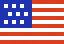New Swift-BAT/GUANO and IceCube Notice Types Available! See news and announcements

# GCN Circular 22513

Subject
Konus-Wind observation of GRB 180314A
Date
2018-03-16T22:19:52Z (6 years ago)
From
Anastasia Tsvetkova at Ioffe Institute <tsvetkova@mail.ioffe.ru>
``````A. Tsvetkova, S. Golenetskii, R.Aptekar, D. Frederiks, P. Oleynik,
M. Ulanov, D. Svinkin, A. Lysenko, A. Kozlova and T. Cline
on behalf of the Konus-Wind team, report:

The long-duration GRB 180314A
(Swift-BAT detection: D'Avanzo�� et al., GCN 22478;
Ukwatta et al., GCN 22490;
Fermi GBM observation: Bissaldi & Roberts, GCN 22485)
triggered Konus-Wind at T0=2601.957 s UT (00:43:21.957).

The burst light curve shows a single emission episode with a total
duration of ~22 s.
The emission is seen up to ~1.5 MeV.

As observed by Konus-Wind, the burst
had a fluence of 1.47(-0.06,+0.06)x10^-5 erg/cm2,
and a 64-ms peak energy flux, measured from T0+0.490 s,
of 2.61(-1.05,+1.21)x10^-6 erg/cm2/s
(both in the 20 keV - 10 MeV energy range).

The time-integrated spectrum of the burst
(measured from T0 to T0+33.024 s)
is best fit in the 20 keV - 1.5 MeV range
by a power law with exponential cutoff model:
dN/dE ~ (E^alpha)*exp(-E*(2+alpha)/Ep)
with�� alpha = -0.61(-0.16,+0.17),
and Ep = 111(-6,+6) keV (chi2 = 45/60 dof).
Fitting by the GRB (Band) model yields the same alpha and Ep,
and an upper limit on the high energy photon index:
beta < -3.08 (chi2 = 45/59 dof).

The spectrum near the peak count rate
(measured from T0 to T0+0.256 s)
is best fit in the 20 keV - 1.5 MeV range
by a power law with exponential cutoff model
with�� alpha = -0.34(-0.57,+0.84),
and Ep = 303(-89,+161) keV (chi2 = 14/15 dof).
Fitting by the GRB (Band) model yields the same alpha and Ep,
and an upper limit on the high energy photon index:
beta < -2.26 (chi2 = 14/14 dof).

Assuming the redshift z=1.445 (Sbarufatti et al., GCN 22484)
and a standard cosmology model with H_0 = 70 km/s/Mpc,
Omega_M = 0.27, and Omega_Lambda = 0.73,
we estimate the following rest-frame parameters:
the isotropic energy release E_iso is 8.18(-0.34,+0.36)x10^52 erg,
the peak luminosity L_iso is 3.55(-1.42,+1.64)x10^52 erg/s,
and the rest-frame peak energy of the time-integrated spectrum,
Ep,i, is 272(-14,+15) keV.

The Konus-Wind light curve of this GRB is available at
http://www.ioffe.ru/LEA/GRBs/GRB180314_T02601/

All the quoted errors are at the 90% confidence level.
All the quoted values are preliminary.
``````
Looking for U.S. government information and services? Visit USA.gov